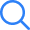# Power Systems T...

TIME LEFT -
• ###### Question 1
2 / -0.33

The ABCD parameters of a 3-phase overhead transmission line are A = D = 0.9 ∠0. B = 200 ∠90° Ω and C = 0.95 × 10-3 ∠90° S. At no-load condition a shunt inductive, reaction is connected at the receiving end of the line to limit the receiving-end voltage to be equal to the sending-end voltage. The ohmic value of the reactor is

• ###### Question 2
2 / -0.33

A power station consists of two synchronous generators of rating 500 MVA and 1000 MVA with inertia 0.8 pu and 0.5 pu respectively on their own buses MVA rating. The equivalent pu inertia constant on 200 MVA common base is:

• ###### Question 3
2 / -0.33

For a delta connected system which of the following statements is true?

• ###### Question 4
2 / -0.33

For a power system network with 10 nodes, Z44 of its bus impedance matrix is j0.5 pu. The voltage at node-4 is 1.5∠2° pu. If a capacitor having a reactance of -j3.5 pu is now added to the network between node-4 and the reference node, the current drawn by the capacitor in pu is _______.

• ###### Question 5
2 / -0.33

A 10-ampere, over current relay having a current setting of 150% and a time setting multiplier of a 0.8 connected to supply circuit through a 400/5 current transformer when the circuit carries a fault current of 5000 A. Find the plug-setting multiplier.

• ###### Question 6
2 / -0.33

Consider the methods given below:

A. Use of additional parallel transmission lines.

B. Upgrading voltage on the existing transmission system.

C. Use of bundled conductors

D. Use of a transformer with lower leakage reactance.

Which of the above methods can be used to improve the steady-state stability limit of a system?

• ###### Question 7
2 / -0.33

The Ybus matrix of a 100-bus interconnected system is 80% sparse. Then, the number of transmission lines in the system must be

• ###### Question 8
2 / -0.33

In a 220 kV, 50 c/s power system, the reactance and capacitance up to the circuit breaker are 8 Ω and 0.025 μF respectively. A resistance of 600 Ω is connected across the circuit breaker contacts. The natural frequency of oscillation is _______ (in kHz)

• ###### Question 9
2 / -0.33

​A three-phase feeder having a resistance of 3 Ω and a reactance of 10 Ω supplies a load of 2 MW at 0.85 lagging power factor. The receiving end voltage is maintained at 11 kV by means of a static condenser drawing 2.1 MVAR from the line. The sending end voltage is

• ###### Question 10
2 / -0.33

Two substations are connected by two lines in parallel with negligible impedance, but each containing a tap changing transformer of reactance 0.18 pu on the basis of its rating of 200 MVA. Find the net absorption of reactive power in MVAr when the transformer taps are set to (1 : 11) and (1 : 0.9) respectively. Assume per unit voltages to be equal at two ends of the substation.

• ###### Question 11
2 / -0.33

A circuit breaker interrupts the magnetizing current of 100 MVA transformer at 220 kV. The magnetizing current of the transformer is 5% of the full load current. The maximum voltage (in volts) which may appear across the gap of the breaker when the magnetizing current is interrupted at 53% of its peak value. The stray capacitance 2500 μF and the inductance is 30 H.

• ###### Question 12
2 / -0.33

A 50 Hz, 4 pole, 500 MVA, 22 kV turbo generator is delivering rated MVA at 0.6 pf. Suddenly a 3.-ph fault occurs at the generator terminal, which will reduce the electrical output by 40% and assume constant power input to the shaft. The accelerating torque of the generator in M(mega) N-m at the fault will be ______ (up to three decimal places)

• ###### Question 13
2 / -0.33

A three phase, 2 pole, 11 kV 10000 kVA alternator has neutral earthed through a resistance of 7 Ω. The machine has current balance protection which operates upon out of balance current that exceed 20% of full load. The % of winding protected against earth fault is _______

Neglect the % reactance of generator winding.

Submit Test##### Question Analysis
• Answered - 0

• Unanswered - 13

• 1
• 2
• 3
• 4
• 5
• 6
• 7
• 8
• 9
• 10
• 11
• 12
• 13
Submit Test×
Open Now